Formulae based

Chapter 2 Class 12 Inverse Trigonometric Functions
Concept wise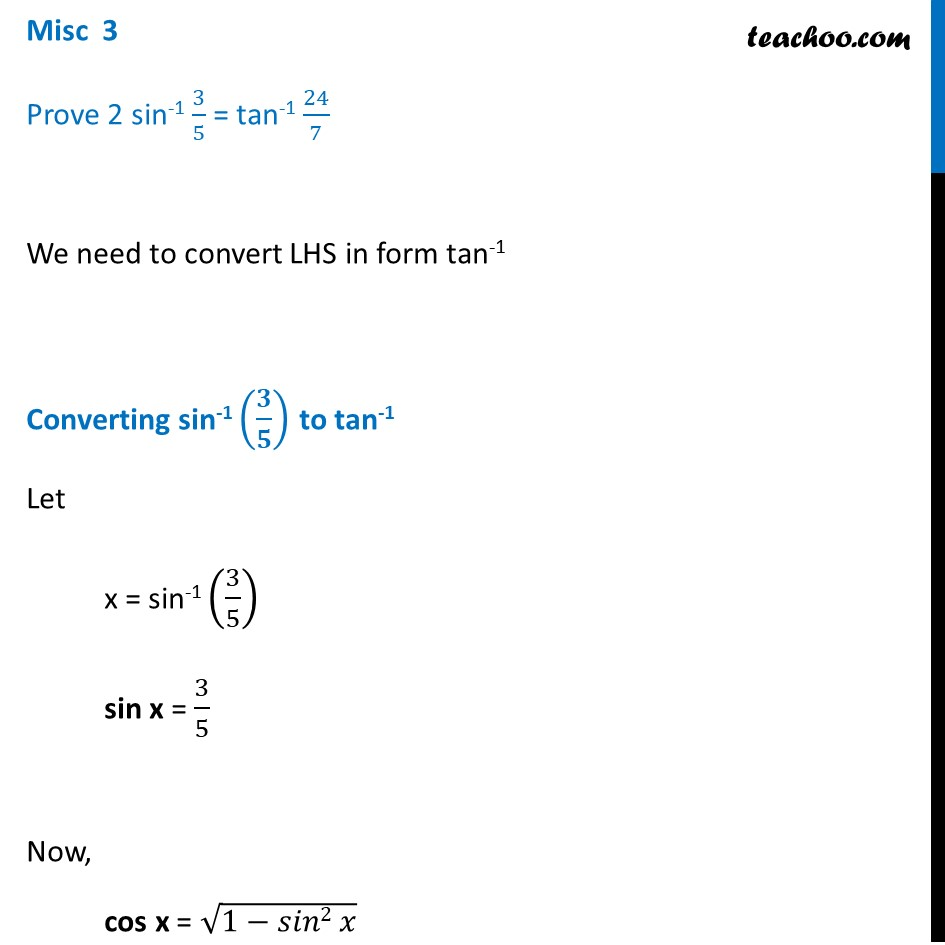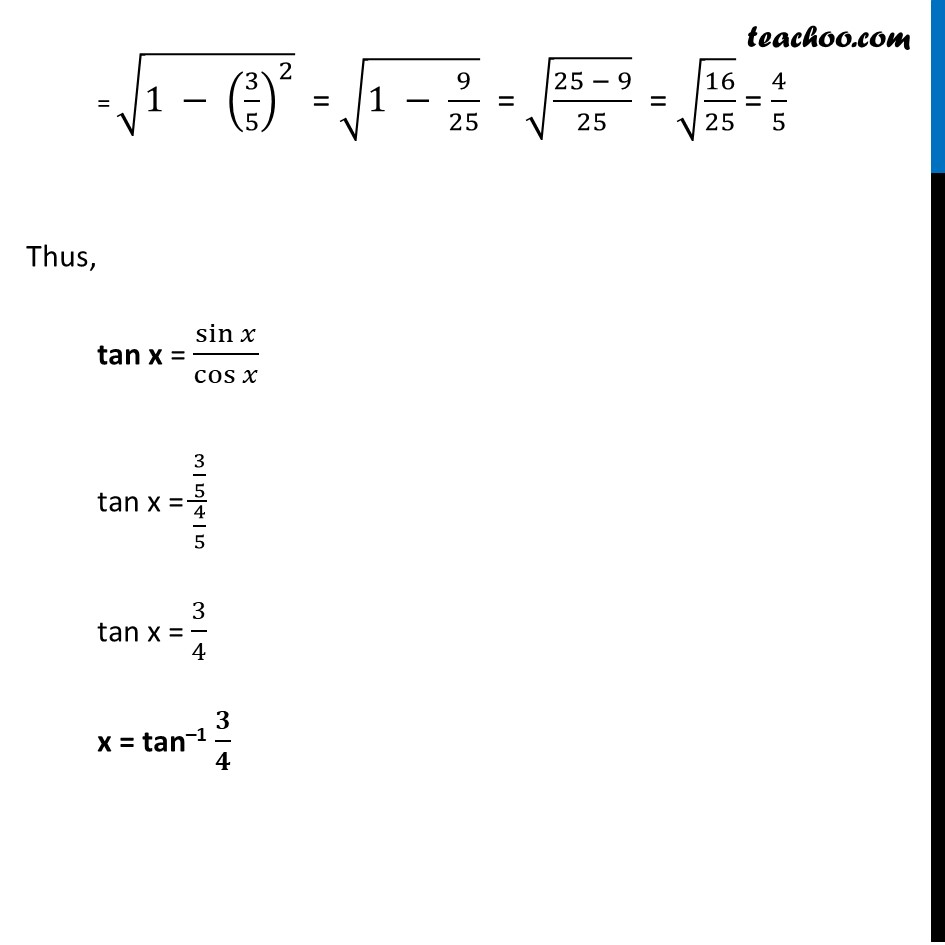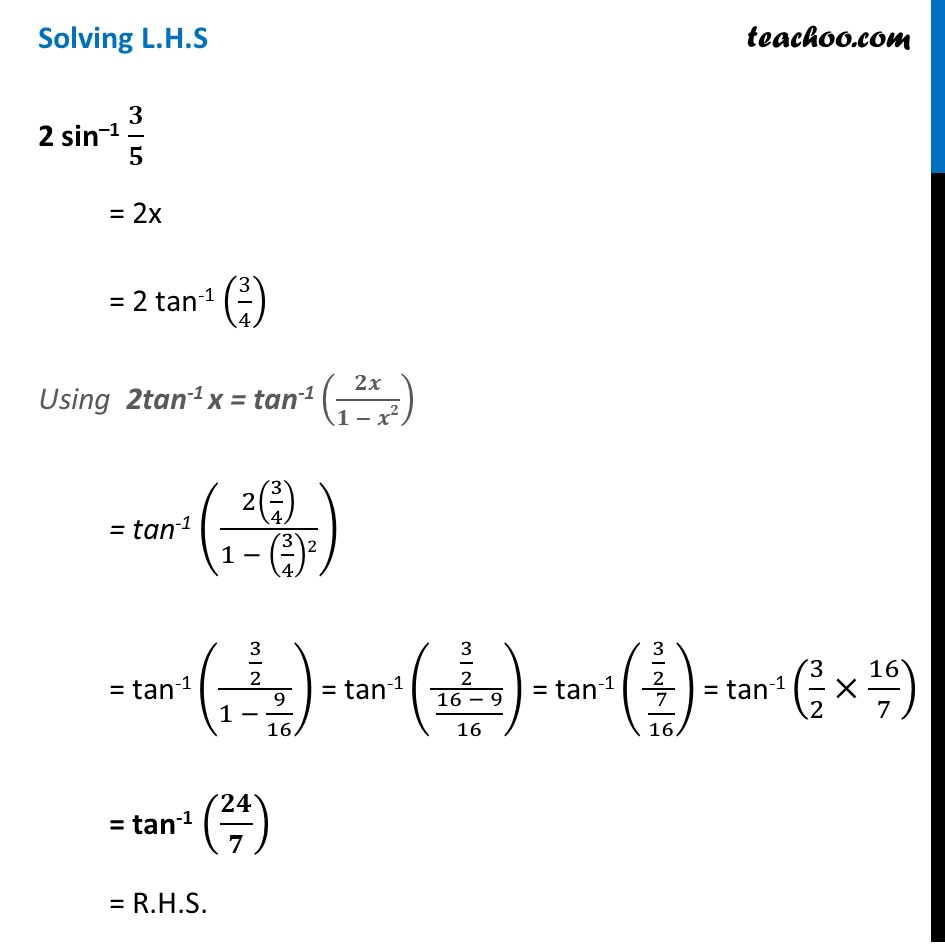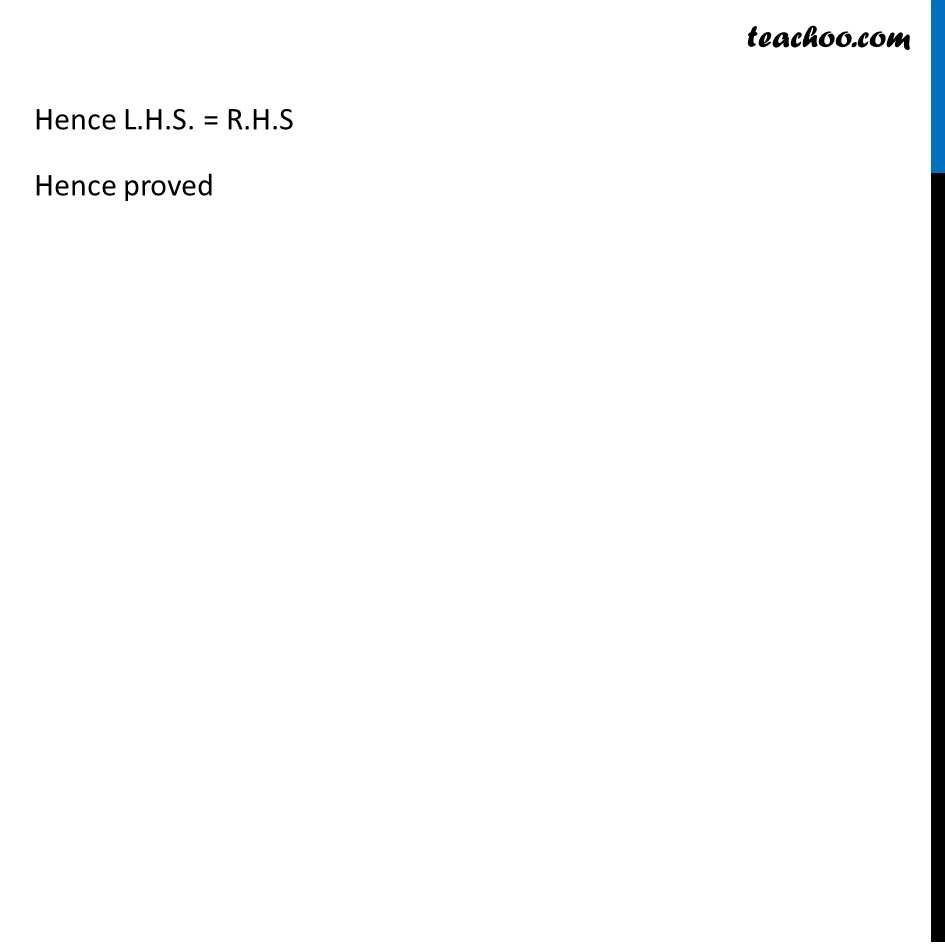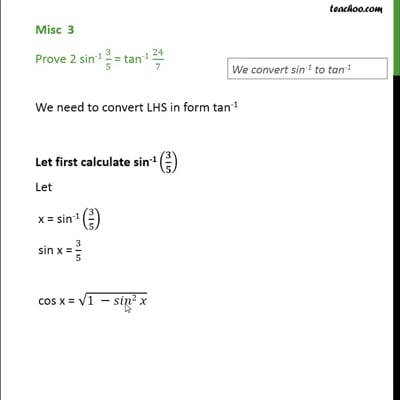This video is only available for Teachoo black users

Solve all your doubts with Teachoo Black (new monthly pack available now!)

### Transcript

Misc 3 Prove 2 sin-1 3/5 = tan-1 24/7 We need to convert LHS in form tan-1 Converting sin-1 (𝟑/𝟓) to tan-1 Let x = sin-1 (3/5) sin x = 3/5 Now, cos x = √(1−𝑠𝑖𝑛2 𝑥) = √(1 − (3/5)^2 ) = √(1 − 9/25) = √((25 − 9)/25) = √(16/25) = 4/5 Thus, tan x = sin⁡𝑥/cos⁡𝑥 tan x = (3/5)/(4/5) tan x = 3/4 x = tan–1 𝟑/𝟒 Solving L.H.S 2 sin–1 𝟑/𝟓 = 2x = 2 tan-1 (3/4) Using 2tan-1 x = tan-1 (𝟐𝒙/(𝟏 − 𝒙𝟐)) = tan-1 (2(3/4)/(1 − (3/4)2)) = tan-1 ((3/2)/(1 − 9/16)) = tan-1 ((3/2)/( (16 − 9)/16)) = tan-1 ((3/2)/( 7/16)) = tan-1 (3/2×16/7) = tan-1 (𝟐𝟒/𝟕) = R.H.S. Hence L.H.S. = R.H.S Hence proved